# Logarithms

Logarithms are used to simplify math.  Multiplication and division can be accomplished through addition and subtraction.

log(1) = 0

log(number greater than 1) = positive

log(number less than 1) = negative

log(A•B) = log(A) + log(B)

log(A/B) = log(A) - log(B)

log(A)n = n•log(A)

# Power ratio expressed in dB

dB stands for decibel (1/10 of a Bel).  The dB is a way of expressing the ratio between two power levels logarithmically.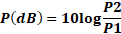P(dB)  =  Numerical power ratio expressed in dB

P1 = Power 1 (input)

P2 = Power 2 (output)

Decibels can be added and subtracted to determine the total gain of a system.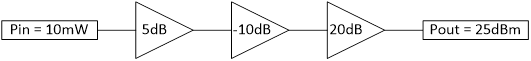Pout = 10dBm + 5dB - 10dB + 20dB = 25dBm

 P(dB) = 10 log10 (P2/P1) Ratio V(dB) = 20 log10 (V2/V1) 120 1.00E+12 240 90 1.00E+09 180 60 1.00E+06 120 50 1.00E+05 100 40 1.00E+04 80 30 1000 60 20 100 40 10 10 20 9.5 9 19.1 9.0 8 18.1 8.5 7 16.9 7.8 6 15.6 7.0 5 14.0 6.0 4 12.0 4.8 3 9.5 3.0 2 6.0 0 1 0 -0.5 0.9 -0.9 -1.0 0.8 -1.9 -1.5 0.7 -3.1 -2.2 0.6 -4.4 -3.0 0.5 -6.0 -4.0 0.4 -8.0 -5.2 0.3 -10.5 -7.0 0.2 -14.0 -10 0.1 -20 -20 0.01 -40 -30 0.001 -60 -40 1.00E-04 -80 -50 1.00E-05 -100 -60 1.00E-06 -120 -90 1.00E-09 -180 -120 1.00E-12 -240

# Power expressed in dBm

Most of the time, power is expressed in dBm in RF applications.  dBm is power relative to one milliwatt.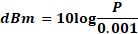dBm Conversion Chart

# Voltage ratio expressed in dB

As long as you use a consistent resistance you can express voltage as a logarithmic ratio as follows: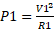and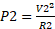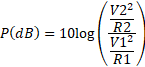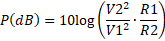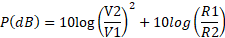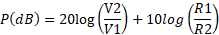When R1 = R2 the second term goes to 0.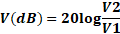V(dB)  =  Numerical voltage ratio expressed in dB

V1 = Voltage 1 (input)

V2 = Voltage 2 (output)

end